# Regularization

The construction of approximate solutions of ill-posed problems that are stable with respect to small perturbations of the initial data (see also Regularization method).

The concept of "regularization" in mathematics is a quite general one, which extends far beyond regularization methods as are used to deal with ill-posed problems. It encompasses at least the following two intermingling ideas.

1) The systematic replacement of a mathematical objectby a more regular one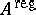, usually in such a way that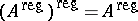.

2) The definition of a value of a function or other concept for objects where that value or concept is a priori undefined (or infinite, undetermined,). This is often done by placing the object in a suitable family (a deformation) in such a way that the function value or concept is defined for all objects in the family near the original one, and then taking a suitable limit. Another technique consists in the removal of "systematic infinities" . The details of various regularization methods that are used depend very much on the particular context. Instead of the word "regularization" , one also finds such methods and techniques labelled by words and phrases like "normalization" , "renormalization" , "desingularization" , "resolution of singularities" ,.

Examples of regularizations in the sense of 1) or 2) above (or both) are: regularized sequences (cf. Regularization of sequences), regularized operators and regularized solutions (cf. Ill-posed problems; Regularization method; Integral equations, numerical methods; Fredholm equation, numerical methods), penalty function and other regularization techniques in optimization theory (cf. Mathematical programming; Penalty functions, method of), various renormalization schemes (cf. Renormalization), the normalization and desingularization of schemes and varieties (cf. Normal scheme; Resolution of singularities), the regularization of distributions (cf. Generalized function), the regularized trace of a Sturm–Liouville operator (cf. Sturm–Liouville problem), and the regularized characteristic determinant of a Hilbert–Schmidt operator.

Still another example is the zeta-function regularization used to define certain (quotients of) infinite determinants in functional integration and quantum field theory. This goes as follows. Letbe a suitable operator, e.g. a Laplace or Laplace–Beltrami operator. Define its generalized zeta-functionwhere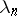runs over the spectrum of(counting multiplicities). At least formally,, which provides the opportunity to try to define the zeta-function regularized determinant by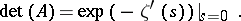For more details (and other related schemes) cf. [a1], [a2].

Two somewhat different uses of the word "regularizing" in mathematics are as follows.

Ifis a bounded linear operator between normed spaces, then a bounded linear operatoris called a "regularizer of a bounded linear operatorregularizer of K" if there are compact operators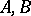such that,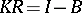. This concept is of importance in the context of singular integral operators, cf. e.g. [a3]. I.e.is an inverse ofmodulo compact operators.

A similar idea, but with deviating terminology, occurs in the theory of pseudo-differential operators. In that context a (pseudo-differential, integral) operator is called regularizing if it takes (extends to an operator that takes) distributions to smooth functions. Given a pseudo-differential operator, an operatoris called a right (left) parametrix ofif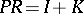(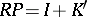), where(respectively,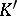) is regularizing; cf. [a4] for a variety of precise statements and results concerning parametrices.